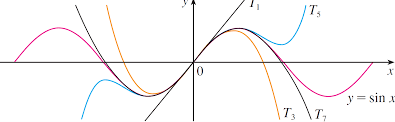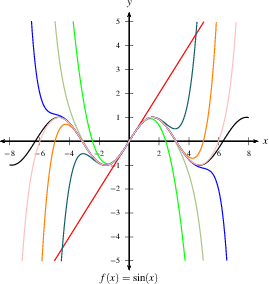Home

#### Integration by Parts

Integration by Parts
Examples
Integration by Parts with a definite integral
Going in Circles

#### Integrals of Trig Functions

Antiderivatives of Basic Trigonometric Functions
Product of Sines and Cosines (mixed even and odd powers or only odd powers)
Product of Sines and Cosines (only even powers)
Product of Secants and Tangents
Other Cases

#### Trig Substitutions

How Trig Substitution Works
Summary of trig substitution options
Examples
Completing the Square

#### Partial Fractions

Introduction to Partial Fractions
Linear Factors
Improper Rational Functions and Long Division
Summary

#### Strategies of Integration

Substitution
Integration by Parts
Trig Integrals
Trig Substitutions
Partial Fractions

#### Improper Integrals

Type 1 - Improper Integrals with Infinite Intervals of Integration
Type 2 - Improper Integrals with Discontinuous Integrands
Comparison Tests for Convergence

#### Modeling with Differential Equations

Introduction
Separable Equations
A Second Order Problem

#### Euler's Method and Direction Fields

Direction Fields
Euler's method revisited

#### Separable Equations

The Simplest Differential Equations
Separable differential equations
Mixing and Dilution

#### Models of Growth

Exponential Growth and Decay
The Zombie Apocalypse (Logistic Growth)

#### Linear Equations

Linear ODEs: Working an Example
The Solution in General
Saving for Retirement

#### Parametrized Curves

Three kinds of functions, three kinds of curves
The Cycloid
Visualizing Parametrized Curves
Tracing Circles and Ellipses
Lissajous Figures

#### Calculus with Parametrized Curves

Video: Slope and Area
Video: Arclength and Surface Area
Summary and Simplifications
Higher Derivatives

#### Polar Coordinates

Definitions of Polar Coordinates
Graphing polar functions
Video: Computing Slopes of Tangent Lines

#### Areas and Lengths of Polar Curves

Area Inside a Polar Curve
Area Between Polar Curves
Arc Length of Polar Curves

#### Conic sections

Slicing a Cone
Ellipses
Hyperbolas
Parabolas and Directrices
Shifting the Center by Completing the Square

#### Conic Sections in Polar Coordinates

Foci and Directrices
Visualizing Eccentricity
Astronomy and Equations in Polar Coordinates

#### Infinite Sequences

Examples of Infinite Sequences
Limit Laws for Sequences
Theorems for and Examples of Computing Limits of Sequences
Monotonic Covergence

#### Infinite Series

Introduction
Geometric Series
Limit Laws for Series
Test for Divergence and Other Theorems
Telescoping Sums

#### Integral Test

Preview of Coming Attractions
The Integral Test
Estimates for the Value of the Series

#### Comparison Tests

The Basic Comparison Test
The Limit Comparison Test

#### Convergence of Series with Negative Terms

Introduction, Alternating Series,and the AS Test
Absolute Convergence
Rearrangements

The Ratio Test
The Root Test
Examples

#### Strategies for testing Series

Strategy to Test Series and a Review of Tests
Examples, Part 1
Examples, Part 2

#### Power Series

Finding the Interval of Convergence
Power Series Centered at $x=a$

#### Representing Functions as Power Series

Functions as Power Series
Derivatives and Integrals of Power Series
Applications and Examples

#### Taylor and Maclaurin Series

The Formula for Taylor Series
Taylor Series for Common Functions
Adding, Multiplying, and Dividing Power Series
Miscellaneous Useful Facts

#### Applications of Taylor Polynomials

Taylor Polynomials
When Functions Are Equal to Their Taylor Series
When a Function Does Not Equal Its Taylor Series
Other Uses of Taylor Polynomials

#### Functions of 2 and 3 variables

Functions of several variables
Limits and continuity

#### Partial Derivatives

One variable at a time (yet again)
Definitions and Examples
An Example from DNA
Geometry of partial derivatives
Higher Derivatives
Differentials and Taylor Expansions

#### Differentiability and the Chain Rule

Differentiability
The First Case of the Chain Rule
Chain Rule, General Case
Video: Worked problems

#### Multiple Integrals

General Setup and Review of 1D Integrals
What is a Double Integral?
Volumes as Double Integrals

#### Iterated Integrals over Rectangles

How To Compute Iterated Integrals
Examples of Iterated Integrals
Fubini's Theorem
Summary and an Important Example

#### Double Integrals over General Regions

Type I and Type II regions
Examples 1-4
Examples 5-7
Swapping the Order of Integration
Area and Volume Revisited

#### Double integrals in polar coordinates

dA = r dr (d theta)
Examples

#### Multiple integrals in physics

Double integrals in physics
Triple integrals in physics

#### Integrals in Probability and Statistics

Single integrals in probability
Double integrals in probability

#### Change of Variables

Review: Change of variables in 1 dimension
Mappings in 2 dimensions
Jacobians
Examples
Bonus: Cylindrical and spherical coordinates

### Taylor Polynomials

Probably the most important application of Taylor series is to use their partial sums to approximate functions.  These partial sums are (finite) polynomials and are easy to compute.  We call them Taylor polynomials.

 An $n^{th}$ degree Taylor polynomial is the polynomial of degree $n$, consisting of the partial sum of the Taylor series up to the $n^{th}$ power, denoted $T_n(x)$: $\begin{eqnarray} T_n(x)&=&f(a)+f'(a)(x-a)+\frac{f''(a)}{2!}(x-a)^2+\frac{f^{(3)}(a)}{3!}(x-a)^3+\cdots+\frac{f^{(n)}(a)}{n!}(x-a)^n\\ &=&\sum_{k=0}^n\frac{f^{(k)}(a)}{k!}(x-a)^k \end{eqnarray}$

We look at various Taylor polynomials:
$\begin{eqnarray} T_1(x)&=&f(a) + f'(a) (x-a)\\ T_2(x)&=&f(a)+f'(a)(x-a)+\frac{f''(a)}{2!}(x-a)^2\\ T_3(x)&=&f(a)+f'(a)(x-a)+\frac{f''(a)}{2!}(x-a)^2+\frac{f^{(3)}(a)}{3!}(x-a)^3\\ &\vdots&\\ T_n(x)&=&f(a)+f'(a)(x-a)+\frac{f''(a)}{2!}(x-a)^2+\frac{f^{(3)}(a)}{3!}(x-a)^3+\cdots+\frac{f^{(n)}(a)}{n!}(x-a)^n \end{eqnarray}$

You may recognize the first Taylor polynomial above.  (Does it look familiar?  Take a moment to think about this.)  It is the linearization $L(x)$ of $f(x)$, which is just the equation of the tangent line of $f$ at $x=a$ that we used previously to approximate values of $f$ near $x=a$: $\quad f(x) \approx L(x) = f(a) + f'(a) (x-a)=T_1(x).$

Taylor polynomials extend the idea of linearization.  To approximate $f$ at a given value of $x$, we will use $T_n(x)$ for a value of $n$ that gives a good enough approximation.  We see from $T_n(x)$ above that we will need to find an $x$-value $a$ at which we can evaluate the derivatives of $f$.  Our approximations get better the more terms we include, and they also allow us to get further from $a$ and still have a good approximation.

Example:  Find the Taylor polynomial of degree $7$ for $f(x)=\sin x$ centered at $x=0$.

Solution:  We will compute this as if we didn't already know the Taylor series for sine.  We look at $T_n(x)$ above, and see we need to find evaluate $f(x)=\sin(x)$ and its derivatives at $a=0$.

$\begin{array}{lll} f(0)&=\sin(0)&=0\\ f'(0)&=\cos(0)&=1 \quad\text{ and then this pattern repeats, so that }\\ f''(0)&=-\sin(0)&=0\\ f^{(3)}(0)&=-\cos(0)&=-1\\ \end{array}$ $\begin{array}{ll} f^{(4)}(0)&=0\\ f^{(5)}(0)&=1\\ f^{(4)}(0)&=0\\ f^{(5)}(0)&=-1\\ \end{array}$

Then $\displaystyle T_7(x)=0+1x+0+\frac{-1}{3!}x^3+0+\frac{1}{5!}x^5+0+\frac{-1}{7!}x^7=x-\frac{x^3}{3!}+\frac{x^5}{5!}-\frac{x^7}{7!}$.  This is, of course, the first few terms of the Taylor Series for $\sin x$.  If you know a series, finding an $n^{th}$ degree Taylor polynomial is easy.  If you don't have the series at hand, you can compute the polynomial as we just did for sine.

My calculator approximates $\sin(.5)=.4794255386$, and $T_7(.5)=.5-\frac{.5^3}{6}+\frac{.5^5}{120}-\frac{.5^7}{5040}=.4794255332$.

 This graphic shows $f(x)=\sin(x)$ in red, then the linear approximations $T_1(x)$, $T_3(x)$, $T_5(x)$ and $T_7(x)$.  You can see that as $n$ gets larger, $T_n(x)$ matches the sine curve better, and matches it farther from 0. cims.nyu.eduThis is another graph of the same function, $f(x)=\sin(x)$, in black.  This graph includes (unlabled) the Taylor polynomials that are in the graph above, with the addition of $T_9(x),T_{11}(x)$, and $T_{13}(x)$.This video will compute approximations of $e^x$ and $\ln(x)$ near $a=0$, and $\sin x$ near $a=\frac{\pi}{4}$.